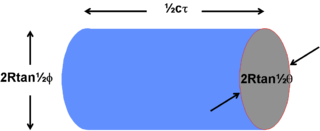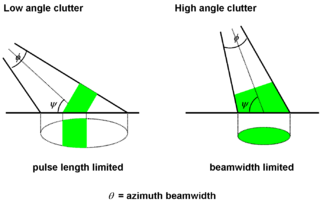﻿

Clutter is a term used for unwanted echoes in electronic systems, particularly in reference to radars. Such echoes are typically returned from ground, sea, rain, animals/insects, chaff and atmospheric turbulences, and can cause serious performance issues with radar systems.

## Backscatter coefficient

What is considered to be clutter by one user may be a target for another. Usually targets may be considered to be a point scatterer and clutter as extended, covering many range, angle and Doppler cells. The clutter may fill a volume (rain) or be confined to a surface (land). In principle all that is required to estimate the return (backscatter) is a knowledge of the volume or surface illuminated and the echo per unit volume, η, or per unit surface area, σ°, (the backscatter coefficient).

## Clutter or Noise limited radar

In addition to any possible clutter there will also always be noise. The total signal competing with the target return is thus clutter plus noise. In practice there is often either no clutter or clutter dominates and the noise can be ignored. In the first case the radar is said to be Noise Limited, in the second it is Clutter Limited.

## Volume Clutter

Rain, hail, snow and chaff are examples of volume clutter. An airborne target, at range R, is within a rainstorm. What is the effect on the detectability of the target?Figure 1. Illustration of illuminated Rain Cell

First find the magnitude of the clutter return. Assume that the clutter fills the cell containing the target, that scatterers are statistically independent and that the scatterers are uniformly distributed through the volume. The clutter volume illuminated by a pulse can be calculated from the beam widths and the pulse duration, Figure 1. If c is the velocity of light and τ is the time duration of the transmitted pulse then the pulse returning from a target is equivalent to a physical extent of cτ, as is the return from any individual element of the clutter. The azimuth and elevation beamwidths, at a range R, are θ / 2 and ϕ / 2 respectively if the illuminated cell is assumed to have an elliptical cross section.

The volume of the illuminated cell is thus:$\ V_m=\pi R \tan(\theta/2)R \tan(\phi/2)(c\tau/2)$

For small angles this simplifies to:$\ V_m\approx\frac{\pi}{4}(R\theta)(R\phi)(c\tau/2)$

The clutter is assumed to be a large number of independent scatterers that fill the cell containing the target uniformly. The clutter return from the volume is calculated as for the normal radar equation but the radar cross section is replaced by the product of the volume backscatter coefficient, η, and the clutter cell volume as derived above. The clutter return is then$\ C=\frac{P_tG_tA_r}{(4\pi)^2R^4}\frac{\pi}{4}(R\theta)(R\phi)(c\tau/2)\eta$ Watts

where

• Pt = transmitter power (Watts)
• Gt = gain of the transmitting antenna
• Ar = effective aperture (area) of the receiving antenna
• R = distance from the radar to the target

A correction must be made to allow for the fact that the illumination of the clutter is not uniform across the beamwidth. In practice the beam shape will approximate to a sinc function which itself approximates to a Gaussian function. The correction factor is found by integrating across the beam width the Gaussian approximation of the antenna. The corrected back scattered power is$\ C=\frac{P_tG_tA_r}{2\log2(4\pi)^2R^4}\frac{\pi}{4}(R\theta)(R\phi)(c\tau/2)\eta$ Watts

A number of simpliflying substitutions can be made. The receiving antenna aperture is related to its gain by:$\ A_r=\frac{G\lambda^2}{4\pi}$

and the antenna gain is related to the two beamwidths by:$\ G=\frac{\pi^2}{\theta\phi}$

The same antenna is generally used both for transmission and reception thus the received clutter power is:$\ C=\frac{P_tG^2\lambda^2}{1024(\log2)\pi^2R^2}c\tau\eta$ Watts

If the Clutter Return Power is greater than the System Noise Power then the Radar is clutter limited and the Signal to Clutter Ratio must be equal to or greater than the Minimum Signal to Noise Ratio for the target to be detectable.

From the radar equation the return from the target itself will be$\ S=\frac{P_tG^2\lambda^2}{(4\pi)^3R^4}\sigma$ Watts

with a resulting expression for the signal to clutter ratio of$\ \frac{S}{C} = \frac{1024(\log2)G\sigma}{(4\pi)^3R^2c\tau\eta}$

The implication is that when the radar is noise limited the variation of signal to noise ratio is an inverse fourth power. Halving the distance will cause the signal to noise ratio to increase (improve) by a factor of 16. When the radar is volume clutter limited, however, the variation is an inverse square law and halving the distance will cause the signal to clutter to improve by only 4 times.

Since$\ G=\frac{\pi^2}{\theta\phi}$

it follows that$\ \frac{S}{C} = \frac{16(\log2)G\sigma}{(4\pi)^3R^2\theta\phi c\tau\eta}$

Clearly narrow beamwidths and short pulses are required to reduce the effect of clutter by reducing the volume of the clutter cell. If pulse compression is used then the appropriate pulse duration to be used in the calculation is that of the compressed pulse, not the transmitted pulse.

### Problems in calculating Signal to Volume Clutter Ratio

A problem with volume clutter, e.g. rain, is that the volume illuminated may not be completely filled, in which case the fraction filled must be known, and the scatterers may not be uniformly distributed. Consider a beam 10o in elevation. At a range of 10 km the beam could cover from ground level to a height of 1750 metres. There could be rain at ground level but the top of the beam could be above cloud level. In the part of the beam containing rain the rainfall rate will not be constant. One would need to know how the rain was distributed to make any accurate assessment of the clutter and the signal to clutter ratio. All that can be expected from the equation is an estimate to the nearest 5 or 10 dB.

## Surface clutter

The surface clutter return depends upon the nature of the surface, its roughness, the grazing angle (angle the beam makes with the surface), the frequency and the polarisation. The reflected signal is the phasor sum of a large number of individual returns from a variety of sources, some of them capable of movement (leaves, rain drops, ripples) and some of them stationary (pylons, buildings, tree trunks). Individual samples of clutter vary from one resolution cell to another (spatial variation) and vary with time for a given cell (temporal variation).

### Beam FillingFigure 2. Illustration of High and Low Angle Surface Clutter Illumination

For a target close to the Earth's surface such that the earth and target are in the same range resolution cell one of two conditions are possible. The most common case is when the beam intersects the surface at such an angle that the area illuminated at any one time is only a fraction of the surface intersected by the beam as illustrated in Figure 2.

### Pulse Length Limited Case

For the pulse length limited case the area illuminated depends upon the azimuth width of the beam and the length of the pulse, measured along the surface. The illuminated patch has a width in azimuth of$\ 2R\tan\theta /2$.

The length measured along the surface is$\ (c\tau/2)\sec\psi$.

The area illuminated by the radar is then given by$\ A = 2R(c\tau/2)(\tan\theta/2)\sec\psi$

For 'small' beamwidths this approximates to$\ A = R(c\tau/2)\theta\sec\psi$

The clutter return is then$\ C=\frac{P_tG^2\lambda^2}{(4\pi)^3R^4}A\sigma^o$ Watts

Substituting for the illuminated area A$\ C=\frac{c}{2^7\pi^3}\frac{P_tG^2\lambda^2}{R^3}\tau\theta\sec\psi\sigma^o$ Watts

where σo is the back scatter coefficient of the clutter. Converting θ to degrees and putting in the numerical values gives$\ C=1300\frac{P_tG^2\lambda^2}{R^3}\tau\theta^o\sec\psi\sigma^o$ Watts

The expression for the target return remains unchanged thus the signal to clutter ratio is$\ \frac{S}{C}=\frac{1}{1300}\frac{R^3}{P_tG^2\lambda^2}\frac{1}{\tau\theta\sec\psi\sigma^o}\frac{P_tG^2\lambda^2}{(4\pi)^3R^4}\sigma$ Watts

This simplifies to$\ \frac{S}{C}=4\times10^{-7}\frac{\cos\psi}{R\tau\theta}\frac{\sigma}{\sigma^o}$

In the case of surface clutter the signal to clutter now varies inversely with R. Halving the distance only causes a doubling of the ratio (a factor of two improvement).

### Problems in calculating clutter for the Pulse length Limited Case

There are a number of problems in calculating the signal to clutter. The clutter in the main beam is extended over a range of grazing angles and the backscatter coefficient depends upon grazing angle. Clutter will appear in the antenna sidelobes, which again will involve a range of grazing angles and may even involve clutter of a different nature.

### Beam Width Limited case

The calculation is similar to the previous examples, in this case the illuminated area is$\ A=\pi R^2\tan^2\theta/2$

which for small beamwidths simplifies to$\ A \approx\pi R^2\theta^2/4$

The clutter return is as before$\ C=\frac{P_tG^2\lambda^2}{(4\pi)^3R^4}A\sigma^o$ Watts

Substituting for the illuminated area A$\ C=\frac{P_tG^2\lambda^2}{(4\pi)^3R^4}\pi R^2(\theta/2)^2\sigma^o$ Watts

This can be simplified to:$\ C=\frac{P_tG^2\lambda^2}{4^4\pi^2R^2}\theta^2\sigma^o$ Watts

Converting θ to degrees$\ C=\frac{P_tG^2\lambda^2}{4^4R^2}(\theta^o/180)^2\sigma^o$ Watts

The target return remains unchanged thus$\ \frac{S}{C}=\frac{4^4R^2}{P_tG^2\lambda^2}(180/\theta^o)^2\frac{1}{\sigma^o}\frac{P_tG^2\lambda^2}{(4\pi)^3R^4}\sigma$

Which simplifies to$\ \frac{S}{C}=5.25\times 10^4\frac{1}{\theta^{o2}R^2}\frac{\sigma}{\sigma^o}$

As in the case of Volume Clutter the Signal to clutter ratio follows an inverse square law.

### General problems in Calculating Surface Clutter

The general significant problem is that the backscatter coefficient cannot in general be calculated and must be measured. The problem is the validity of measurements taken in one location under one set of conditions being used for a different location under different conditions. Various empirical formulae and graphs exist which enable an estimate to be made but the results need to be used with caution.

Wikimedia Foundation. 2010.

### Look at other dictionaries:

• Clutter (Radar) — verschiedene Störungen auf dem Radarschirm Unter Clutter versteht man Ziele, die das Radargerät erfasst und anzeigt, die aber unerwünscht sind, da sie die Entdeckungswahrscheinlichkeit der eigentlichen Ziele herabsetzen (der Begriff ist aus der… …   Deutsch Wikipedia

• Clutter — may refer to any of the following: Excessive physical disorder Clutter (organizing), a confusing or disorderly state or collection, and possible symptom of compulsive hoarding A type of light pollution Clutter (radar), unwanted echoes in… …   Wikipedia

• Clutter — steht für Clutter (Radar), von einem Radargerät erfasste, aber unerwünschte Ziele Clutter (Bibliothek), eine freie Softwarebibliothek zur Entwicklung grafischer Benutzeroberflächen Herbert W. Clutter (1911–1959), Mordopfer, das durch Truman… …   Deutsch Wikipedia

• radar clutter — radiolokatoriaus trukdžiai statusas T sritis Gynyba apibrėžtis Nepageidautini signalai, atspindžiai ar atvaizdai ekrane, trukdantys stebėti reikiamus signalus. atitikmenys: angl. clutter; radar clutter pranc. fouillis; interférence; interférence… …   NATO terminų aiškinamasis žodynas

• clutter — radiolokatoriaus trukdžiai statusas T sritis Gynyba apibrėžtis Nepageidautini signalai, atspindžiai ar atvaizdai ekrane, trukdantys stebėti reikiamus signalus. atitikmenys: angl. clutter; radar clutter pranc. fouillis; interférence; interférence… …   NATO terminų aiškinamasis žodynas

• Radar MASINT — is one of the subdisciplines of Measurement and Signature Intelligence (MASINT) and refers to intelligence gathering activities that bring together disparate elements that do not fit within the definitions of Signals Intelligence (SIGINT),… …   Wikipedia

• Clutter folding — is a term used in describing clutter seen by radar systems. Clutter folding becomes a problem when the range extent of the clutter (seen by the radar) exceeds the pulse repetition frequency interval of the radar, and it no longer provides… …   Wikipedia

• RADAR — On désigne sous le nom de radar (RAdio Detection And Ranging ) un système qui illumine une portion de l’espace avec une onde électromagnétique et reçoit les ondes réfléchies par les objets qui s’y trouvent, ce qui permet de détecter leur… …   Encyclopédie Universelle

• Radar jamming and deception — is the intentional emission of radio frequency signals to interfere with the operation of a radar by saturating its receiver with noise or false information. There are two types of radar jamming: Mechanical and Electronic jamming . Mechanical… …   Wikipedia

• Radar Météorologique — Un radar météorologique est un type de radar utilisé en météorologie pour repérer les précipitations, calculer leur déplacement et déterminer leur type (pluie, neige, grêle, etc.). La structure tridimensionnelle des données obtenues permet… …   Wikipédia en Français